Updated: 10/12/2016

# Hip Biomechanics

Topic
Review Topic
0
0
Questions
5
0
0
Evidence
3
0
0
Videos
1
Cases
1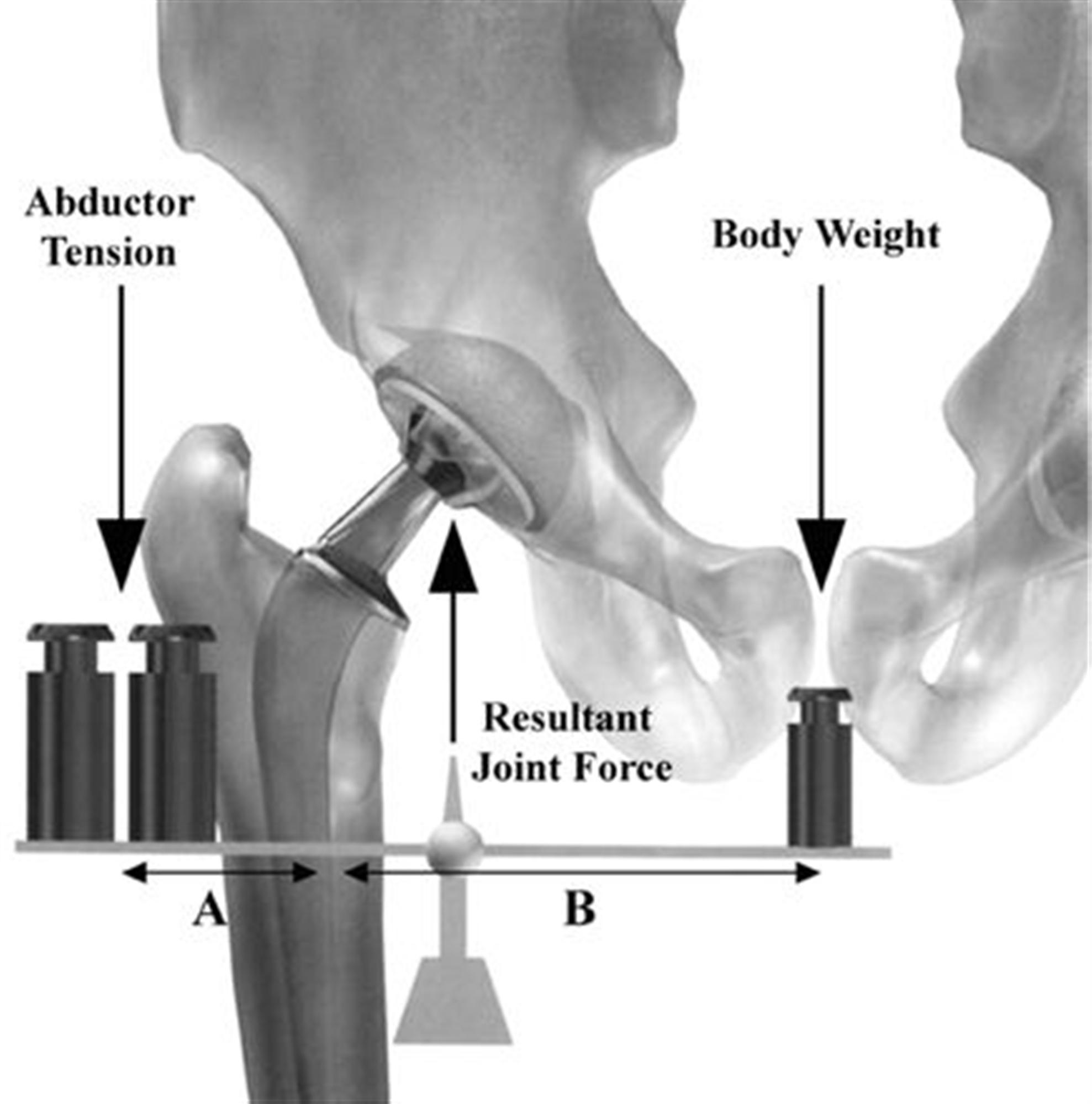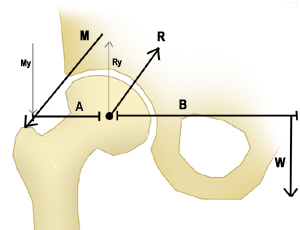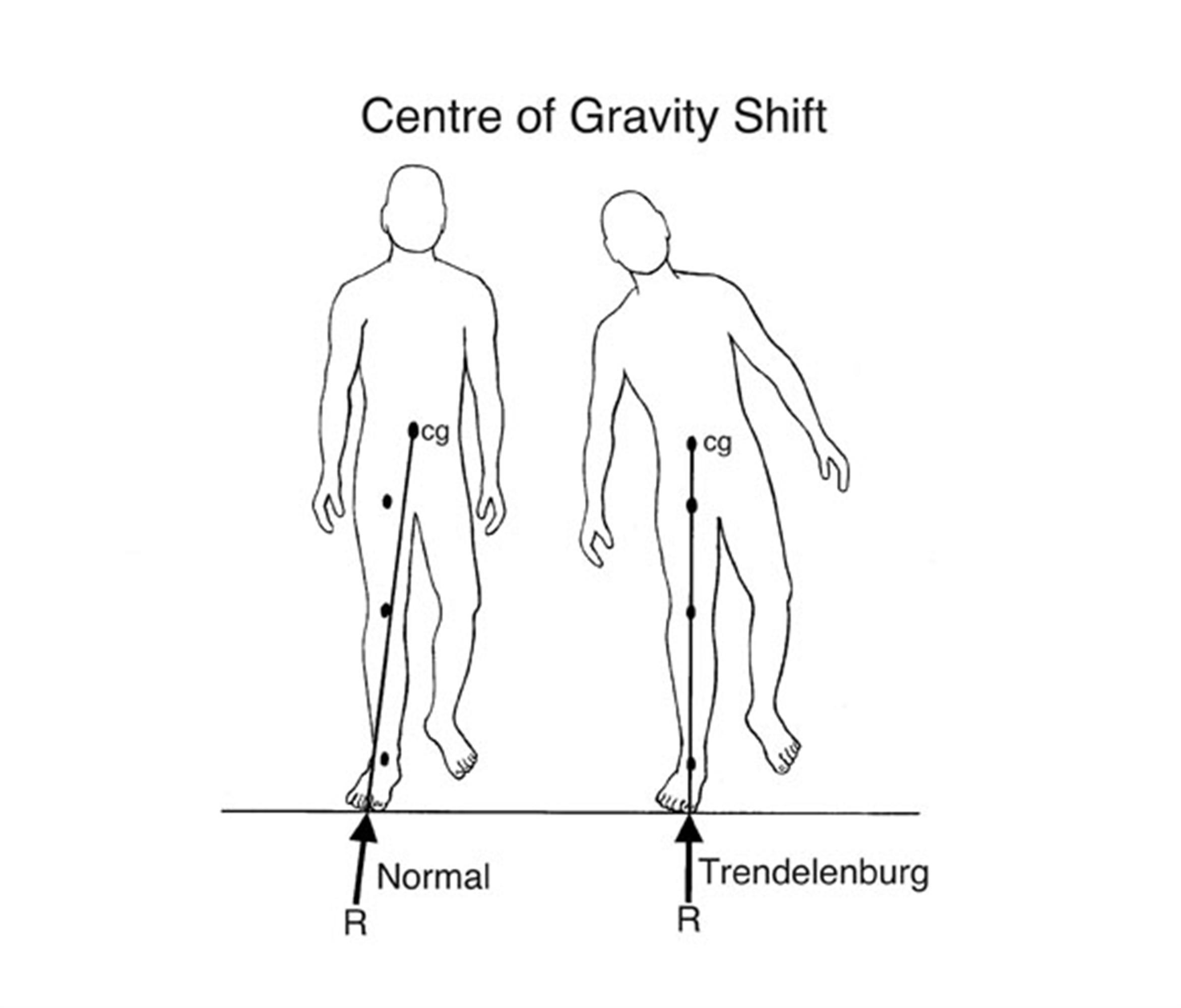Joint Biomechanics Definitions Joint reaction force defined as force generated within a joint in response to forces acting on the joint in the hip, it is the result of the need to balance the moment arms of the body weight and abductor tension (see diagram to right) maintains a level pelvis Coupled forces when two movements and associated forces are coupled Joint congruence relates to fit of two articular surfaces high congruence increases joint contact area Instant center of rotation point about which a joint rotates often changes during rotation due to joint translation center of gravity of human is just anterior to S2 Friction and lubrication not a function of contact area lubrication decreases friction examples coefficient of friction of human joints is .002 to .04 TJA (metal on PE) is .05 to .15 Free Body Analysis Definitions Newtons laws first law if there is no net force on an object, its velocity remains constant second law force equals mass multiplied by acceleration F=ma third law when a first body exerts a force on a second body, the second body exerts a force that is equal in magnitude and opposite in direction on the first body F2=-F1 Force definition a push or pull on an object resulting from the object's interaction with another object equation force = mass x acceleration, F=ma 1 Newton = force required to give 1 kg mass an acceleration of 1 m/s2  Vector definition a quantity that contains both direction and magnitude scalar quantities do not have direction  forces and velocity can be broken down into vectors Moment (torque) definition the tendency of a force to rotate a body around an axis equation moment (torque) = force(perpendicular) X distance Work definition when a force acts upon an object to create displacement equation work = force (vector parallel to displacement) x distance Energy definition ability of an object to perform work classification potential energy equation U (potential energy) = mass x gravity x height kinetic energy equation KE (kinetic energy) = 1/2mv2 Hip Free Body Analysis Free body analysis diagramW = gravitational force weight of the body minus weight of ipsilateral extremity (or 5/6 body weight) M = abductor muscle force R = joint reaction force can reach 3 to 6 times body weight Solving for joint reaction force (R) step 1:  calculate My principle sum of all moments equals 0  in this case, the moments are created by My and W equation  (A x My) + (B x W) = 0 assume A = 5cm and B = 12.5cm (this information will be given to you) My = 2.5W step 2:  calculate Ry Ry = My + W Ry = 2.5W + W Ry = 3.5W step 3:  calculate R R = Ry / (cos 30°) R = 3.5W / (cos 30°) R = ~4W Clinical Implications Actions that decrease joint reaction force include  increase in ratio of A/B (shift center of rotation medially) acetabular side moving acetabular component medial, inferior, and anterior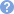femoral side increasing offset of femoral componentlong stem prosthesis lateralization of greater trochanter by using increased offset neck/prosthesis varus neck-shaft angulation increases shear across joint patient's gait shifting body weight over affected hipthis results in Trendelenburg gait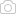cane in contralateral hand  reduces abductor muscle pull and decreases the moment arm between the center of gravity and the femoral headcarrying load in ipsilateral hand  produces additional downward moment on same side of rotational point Actions that increase joint reaction force include valgus neck-shaft angulation decreases shear across joint

Average 3.3 of 38 Ratings

Thank you for rating! Please vote below and help us build the most advanced adaptive learning platform in medicine

The complexity of this topic is appropriate for?
How important is this topic for board examinations?
How important is this topic for clinical practice?
Questions (5)

(OBQ11.221) A cane held in the contralateral hand reduces joint reactive forces through the affected hip approximately 50% by which of the following mechanisms? Review Topic

QID: 3644
1

Reducing hip abductor muscle pull

72%

(2487/3447)

2

Increasing hip flexor muscle pull

1%

(32/3447)

3

Moving the center of rotation for the femoroacetabular joint

22%

(750/3447)

4

Increasing joint congruence at the femoroacetabular joint

1%

(32/3447)

5

Moving the center of gravity posterior to the second sacral vertebra

4%

(124/3447)

ML 3

Select Answer to see Preferred Response

PREFERRED RESPONSE 1Sorry, this question is for
PEAK Premium Subscribers onlySorry, this question is for
PEAK Premium Subscribers only

(OBQ09.172) Figure A represents a free body diagram of the hip of a patient standing on the right leg. The forces and distances are labeled on the diagram and the resulting hip joint force (J) = 1800N. What is the resultant value for J when the acetabular component is medialized given the new distances shown in Figure B? Review Topic

QID: 2985
FIGURES:
1

1000N

6%

(126/2255)

2

1200N

63%

(1413/2255)

3

1800N

8%

(170/2255)

4

2200N

11%

(241/2255)

5

3600N

12%

(265/2255)

ML 3

Select Answer to see Preferred Response

PREFERRED RESPONSE 2

(OBQ05.76) Patients display a Trendelenburg gait to compensate for weakness in which of the following muscle groups? Review Topic

QID: 962
1

1%

(10/1157)

2

hip abductors

98%

(1129/1157)

3

hip flexors

1%

(7/1157)

4

knee extensors

0%

(1/1157)

5

hip extensors

1%

(6/1157)

ML 1

Select Answer to see Preferred Response

PREFERRED RESPONSE 2
ARTICLES (8)
VIDEOS (1)
CASES (1)